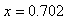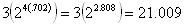Method 2
Example 2

Solve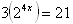Step 1. Isolate the exponential expression. First, divide both sides by 3.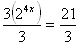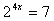Step 2. Since there is no common base, apply logarithms.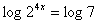Step 3. Use the Power to a Power Property.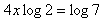Step 4. Solve for x.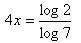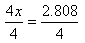Step 5. Use a calculator to find the answer.